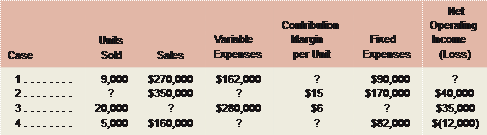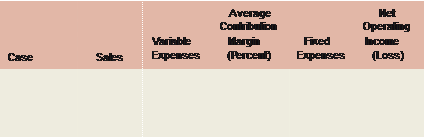### Create an Account

Home / Questions / Missing Data Basic CVP Concepts Fill in the missing amounts in each of the eight case sit...

# Missing Data Basic CVP Concepts Fill in the missing amounts in each of the eight case situations below. Each case is independent of the others Hint One way to find the missing amounts would be

Missing Data; Basic CVP Concepts

Fill in the missing amounts in each of the eight case situations below. Each case is independent of the others. (Hint: One way to find the missing amounts would be to prepare a contribution format income statement for each case, enter the known data, and then compute the missing items.)

a.       Assume that only one product is being sold in each of the four following case situations:b.Assume that more than one product is being sold in each of the four following case situations:

 1 . . . . . . . \$450,000 ? 40% ? \$65,000 2 . . . . . . . \$200,000 \$130,000 ? \$60,000 ? 3 . . . . . . . ? ? 80% \$470,000 \$90,000 4 . . . . . . . \$300,000 \$90,000 ? ? \$(15,000)Jun 28 2020 View more View LessSubscribe To Get Solution